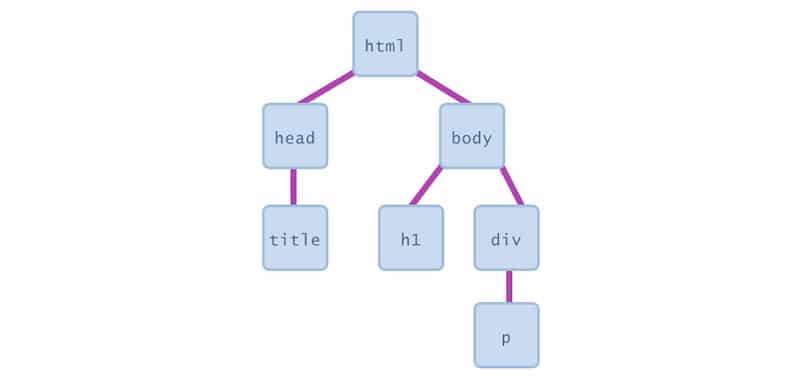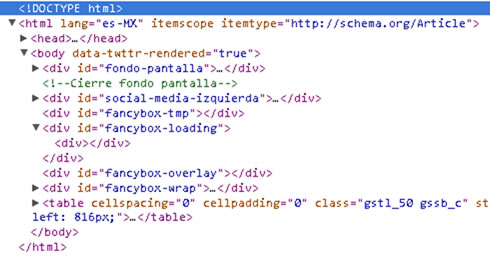# JavaScript DOM Document Object Model - Events and operators

JavaScript considers each of the elements of the tree as objects. The objects within the document are called the DOM Document Object Model.## DOM, events, values, arithmetic and comparison operators

In a Web page, the objects that make up the page (or document) are represented by a tree structure.

You will have seen this kind of thing before creating HTML pages, the top level of the page is inside the and tags, along with other tags inside each of them, and so on.

Some browsers can show a representation of this tree structure.With the DOM inspector, which is part of Google Chrome, you can see the tree structure of the document; Firefox, Safari, and Internet Explorer incorporate similar functions.

JavaScript considers each of the elements of the document tree as objects, and can use JavaScript to manipulate those objects.

The representation of the objects within the document is called Document Object Model (DOM)

Each of the objects in the tree is also called the node of the tree. We can use JavaScript to modify any aspect of the tree, in addition to being able to add, access, modify and remove the nodes from the tree.

Each object of the tree is a node. If the node contains an HTML tag, it receives the name node element; otherwise, it is called a text node. Of course, the element nodes can contain text nodes.

## Event Handling

Events are actions that the user performs while visiting a page. Submitting a form and moving the mouse over an image are two examples of events.

JavaScript uses commands called event handlers to work with events.

A user action on the page triggers an event handler in his script.

In the following table you will find the list of the twelve most common event handlers.

## Events handlers

Events What it handles

Onabort

The user aborted the page load

Onblur

The user left the object

Onchange

The user changed the object

Onerror

The script encountered an error

Onfocus

The user activated an object

Onmouseover

The cursor moved on an object

Onmouseout

The cursor was removed from an object

Onselect

The user selected the content of an object

Onsubmit

The user sent a form

The user left the page

Let's say, for example, that our cat manipulates the event onmimo performing the actions purr and stretch.

In JavaScript, if the user clicks a button, the onclick event handler will record the action and perform the assigned tasks.

When writing a script, it is not necessary to anticipate any possible action that the user can take, enough with those in which you want something special to happen.

For example, your page will load well without the need for an onLoad event handler. But you should use the onLoad command if you want to launch a script as soon as the page loads.

## Values and variables

In JavaScript, a piece of information is a value. There are different kinds of values; the ones that will be most familiar to you are the numbers.

A string value is a word or words enclosed in quotation marks.

The following table shows a list of JavaScript values.

### Types of values

Type Description Example

Number

Any numeric value

3.141592654

String

Characters in quotes

"¡Hello World!"

Boolean

True or false

True

Null

Empty and meaningless

Object

Any value associated with the object

Function

Value returned by a function

The variables contain values. For example, the variable "MyName" can be assigned the string "Aurora".

Another way to write this is my name = "Aurora". The equality sign can be read as "is set to", or as "is equal to".

In other words, the variable myName now contains the value Aurora.

Tips

• JavaScript is case-sensitive. This means that my name is not the same as myName, or MyName
• Variable names cannot contain spaces or other punctuation characters, not starting with a digit. Neither can any of the JavaScript reserved words be used

## Operators in JavaScript

Operators are the symbols used to work with variables.

He is already familiar with them thanks to elementary arithmetic: the plus and minus signs are operators.

The following table shows the complete list of operators.

### Operators

Number Any numerical value

x+y (numerical)

X and Y sum

x+y (string)

Concatenate xy

x-y

Subtract y from x

x*y

Multiply x y

x/y

Divide x between y

X % y

Module xy (for example, the rest when x is divided by y)

X++, ++x

Add one ax (same as x = x + 1)

X--,--x

Subtract one from x (same as x = x-1)

-x

Reverse the sign of x

Tips
Although both x ++ and ++ x add one to the variable x, they are not identical; the first increases x after the assignment has been completed, and the second, before. For example, if x is 5, y = x ++ results in y being set to 5, y = x ++ results in y being set to 5 yxa 6, while y = ++ x results in both x and y and assigned the value 6. The operator - (minus sign) works in a similar way.

## Assignments and comparisons

When you place a value in a variable, that value is assigned to the variable, and for this you must use an assignment operator.

For example, use the equality operator to make an assignment, as in the case of yourName = "Antonio".

The full list of assignment operators is shown in the following table.

### Assignment

Assignment Action

X=y

Set x to the value of y (assign the value of yax)

X+=y

Equal to x = x + y

x-=y

Equal to x = x and

X*=y

Equal to x = x * y

x/=y

Same as x = x / y

X%=y

Same as x = x

Assignment operators other than the equality sign serve as shortcuts to modify the value of the variables. For example, a shorter way to say x = x + 5 is x + = 5

## Comparisons

You will often have to compare the value of one variable with another, or the value of a variable with a literal value (that is, the value directly written in the expression).

For example, you could compare the value of the day of the week with "Tuesday", for which you will have to check if todayDate == "Tuesday".

The complete list of comparisons is shown in the following table.

### Comparisons

Comparison Action

X==y

Returns true if xy are equal

X!=y

Returns true if xy are not equal

x>y

Returns true if x is greater than y

x>=y

Returns true if x is greater than or equal to y

X<y

Returns true if x is less than y

X<=y

Returns true if x is less than or equal to y

X&&y

Returns true if xy are true

X||y

Returns true if x or y is

!x

Returns true if x is false

Tip
If you are comparing strings, keep in mind that "a" is greater than "A", and that "abracadabra" is better than "be".

## Did you like it or was it useful?

Help us share it in Social Networks

Professor at the University of Guadalajara

Professional in Web Development and SEO Positioning for more than 10 continuous years.
We have more than 200 certificates and recognitions in the Academic and Professional trajectory, including diploma certificates certified by Google.

## IT ALSO DESERVES TO PAY TO VISIT:

### PREVIOUS

Objects, Properties and Methods in JavaScript